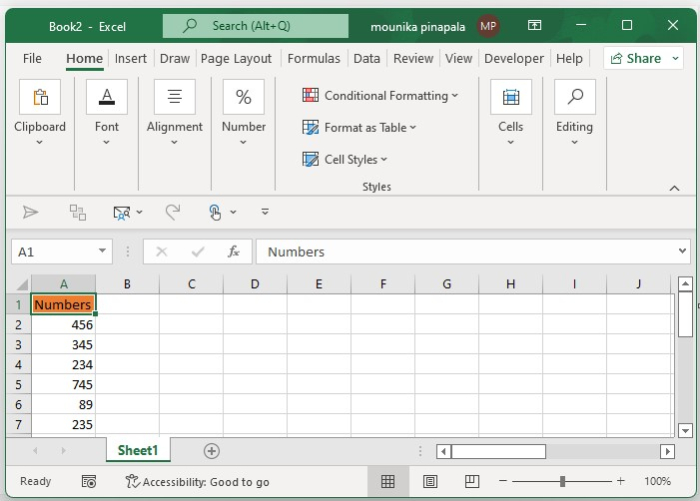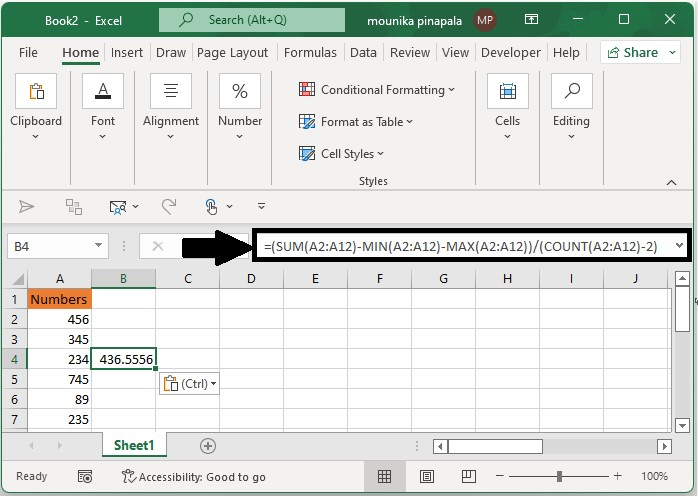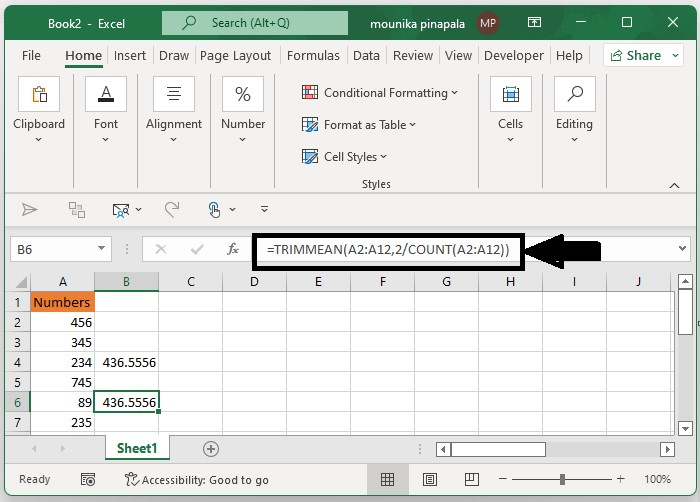# How to calculate average without max and min values in Excel?

You know how to calculate the normal average function which gives the average value of all the selected cells in an excel sheet. But in this article, you will learn how to calculate the average excluding the min and max values or highest and lowest values from selected numbers as shown below.

You can calculate the average without max and min values in Microsoft excel using the formulas.

Open a Microsoft excel sheet and enter the numbers as per your need as shown in the below screenshot for your reference.Now you need to enter the below-given formula and then press enter key which can help you to average the range of values without the max and min values or numbers as shown in the below screenshot for your reference.

=(SUM(A2:A12)-MIN(A2:A12)-MAX(A2:A12))/(COUNT(A2:A12)-2)You can also use the below given formula to calculate the average without the min and max values which gives the same result as the above formula. Kindly find the below given screenshot for your reference.

=TRIMMEAN(A2:A12,2/COUNT(A2:A12))Note − In the given formula, A2:A12 is the range of numbers that you want to calculate you can also change these numbers as per your need.

The result obtained is the same, 436.5556 as shown in the above screenshots, when you use the above two formulas given formulas in a Microsoft excel sheet.

This is the easiest method and quick method to find the average without a max and min value or highest value or lowest value in a Microsoft excel sheet.

## Conclusion

In this tutorial, we used a simple example to demonstrate how you can calculate the average without max and min values using the formulas in an Excel sheet.